# Excel IF formula with operator : greater than,less than

This post will guide you how to use if function with “greater than”,  “greater than or equal to”, ”less than” and “less than or equal to” logical operators in excel.

## Greater than, Greater than or equal to, Less than, less than or equal to

The “greater than”(>)operator will compare the size of two different values in cells and then return TRUE if the first value in cells compared is larger than the second values in cells. FALSE if they are not.

The “greater than or equal to” (>=) operator will return TRUE if the first value in cells is larger than the second or if the two values are equal.

The “Less than” operator returns TRUE if the first value in cell is smaller than the second value in cells.

The “Less than or equal to” operator returns TRUE if the first value in cell is smaller than the second of the two values are equal.

Those excel comparison operators mostly used to compare numbers, date and time values.

Also, we can use those operators to compare text string, and it will compare the value of the first letters in the text string. And if the first letters are same, it will compare the second letters and so on.

Let’s see the below excel if formula with greater than operator:

 Formula example Description =A1>10 Returns True if a number value in cell A1 is greater than 10, FALSE if they are not. =A1>=(B1*2) Returns True if a number value in cell A1 is greater than or equal to the value of  B1*2, FALSE if they are not. =A1

## The Generic IF formula with Greater than operator

Now we can use the above logical test with greater than operator. Let’s see the below generic if formula with greater than operator:

`=IF(A1>10,”excelhow.net”,”google”)`

If you want to take the different action when a value in cells is greater than a certain value (it can be number, text, date or another function), then you can use the IF function with greater than operator to make a logical test and return one value if the logical test condition is true, or return another value if the logical test condition is false.

For the above IF formula, it just tests the value in cell A1 to check if the value is greater than 10. If TRUE, the IF formula will return “excelhow.net”. If FALSE, the IF function will return “google”.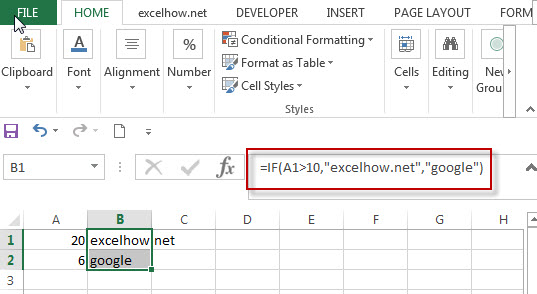You might want to return “excelhow.net” if the test condition is TRUE. Or return nothing if the test returns FALSE. You can use an empty string (“”) as False value in the IF function, see below if formula:

`=IF(A1>10,”excelhow.net”,””)`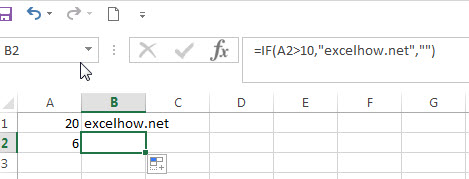## The Generic IF formula with Greater than or equal to operator

`=IF(A1>=(B1*2),”excelhow.net”,”google”)`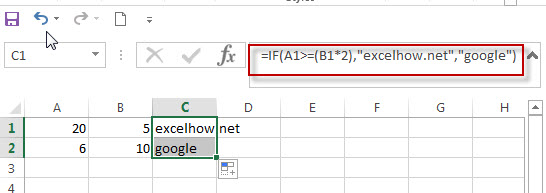## The Generic IF formula with less than operator

`=IF(A1<DATEVALUE(“10/30/2018”),”excelhow.net”,”google”)`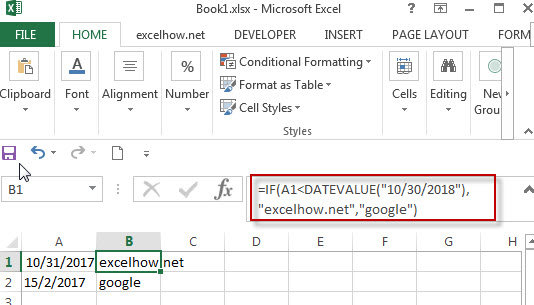## The Generic IF formula with Greater than operator

`=IF(A1<=”excelhow”,”excelhow.net”,”google”)`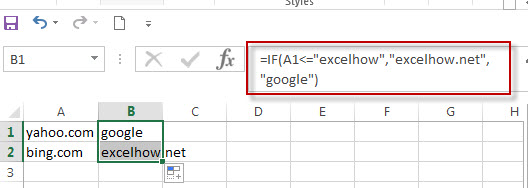### Related Functions

• Excel AND function
The Excel AND function returns TRUE if all of arguments are TRUE, and it returns FALSE if any of arguments are FALSE.The syntax of the AND function is as below:= AND (condition1,[condition2],…) …
• Excel IF function
The Excel IF function perform a logical test to return one value if the condition is TRUE and return another value if the condition is FALSE….
• Excel nested if function
The nested IF function is formed by multiple if statements within one Excel if function. This excel nested if statement makes it possible for a single formula to take multiple actions…
• Excel DATEVALUE Function
The Excel DATEVALUE function returns the serial number of date. And it can be used to convert a date represented as text format into a serial number that recognizes as a date format.The syntax of the DATEVALUE function is as below:=DATEVALUE(date_text)…